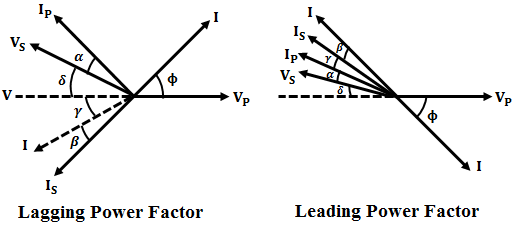# Extension of Range of Wattmeter - Using Instrument Transformers (CT & PT)

In the case of circuits that carry large currents and voltages, the measurement of power can not be carried out by normal wattmeters. Thus, there is a need for an extension of the range of wattmeter. By using instrument transformers (current & potential transformer) in conjunction with a normal wattmeter, the measuring range of the wattmeter can be extended.

## Extension of Range of Wattmeter :

By using a current and potential transformer in conjunction with a wattmeter, high power measurements can be done. The current and potential transformers are connected between the line and current and voltage coils of a wattmeter respectively. The circuit diagram for measuring power using instrument transformers is shown below.

From the above figure, it can be seen that the voltage and current transformers are to be used along with the wattmeter for measurement of power. The primary of the current transformer (CT) is connected in series with the line and the secondary of the CT is connected to the current coil of the wattmeter through an ammeter.

Similarly, the primary of the potential transformer (PT) is connected in parallel to the line and the secondary is connected to the voltage coil of the wattmeter through a voltmeter. These both transformers step down the high voltage and current values to a value measurable by the voltage and current coils of the wattmeter. By knowing the transformation ratios of CT and PT, the power to the load can be determined.

In order to extend the range of the wattmeter, many current transformers and potential transformers of varied ratios are to be used along with the current and voltage coils of the wattmeter respectively. Thereby, a single wattmeter can cover a wide range of power measurements.

## Phasor Diagram & Correction Factor :

Due to the presence of ratio and phase angle errors in CT and PT, it is necessary to consider these errors in order to achieve precise measurement. Hence, the following corrections are made for errors in instrument transformers.

#### Let,V = Voltage of loadI = load currentθ = Phase angle between load current and voltage (power factor)β = Phase angle of CTα = Angle by which current Ip in wattmeter voltage coil lags behind Vs due to its inductanceγ = Phase angle between currents in current and voltage coils of wattmeterδ = Phase angle of PTVs = Voltage applied to wattmeter voltage coilIs = Current in wattmeter current coil The phase angle between the currents in the current and voltage coils of wattmeter is,γ = Φ - α - β ± δ (for lagging pf)γ = Φ + α + β ± δ (for leading pf)The phasor diagrams for leading and lagging power factors are given as follows,If the transformation ratio of CT and PT are neglected. Then, the correction factors in case of lagging pf and leading pf are given as,Hence, the expression for the true power can be written as,True Power = K x Actual ratio of CT x Actual ratio of PT x Wattmeter reading

Do not enter any spam links and messages